Next: About this document ... Up: lab_template Previous: lab_template

Subsections

# Partial Derivatives and their Geometric Interpretation

## Purpose

The purpose of this lab is to acquaint you with using Maple to compute partial derivatives.

## Background

For a function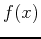of a single real variable, the derivativegives information on whether the graph of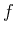is increasing or decreasing. Finding where the derivative is zero was important in finding extreme values. For a function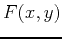of two (or more) variables, the situation is more complicated.

### Partial derivatives

A differentiable function,, of two variables has two partial derivatives: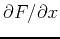and. As you have learned in class, computing partial derivatives is very much like computing regular derivatives. The main difference is that when you are computing, you must treat the variable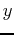as if it was a constant and vice-versa when computing.

The Maple commands for computing partial derivatives are D and diff. The diff command can be used on both expressions and functions whereas the D command can be used only on functions. The examples below show all first order and second order partials in Maple.

> f := (x,y) -> x^2*y^2-x*y;
> diff(f(x,y),x);
> diff(f(x,y),y);
> diff(f(x,y),x,x);
> diff(f(x,y),y,y);
> diff(f(x,y),x,y);
> D(f)(x,y);
> D(f)(x,y);
> D[1,1](f)(x,y);
> D[2,2](f)(x,y);
> D[1,2](f)(x,y);


The next example shows how to evaluate the mixed partial derivative of the function given above at the point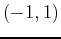.

> der := diff(f(x,y),x,y);
> subs({x=-1,y=1},der);
> D[1,2](f)(-1,1);


## Tangent Lines and Planes

The tangent plane like the tangent line to a single variable function is based on derivatives, however the partial derivatives are used for the tangent plane. Let's start with the equation of the tangent line to the functionat the point where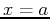. Recall, the general equation of a line at the point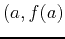having slopeis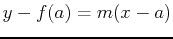. This can be rewritten knowing that the derivative is the slope of a tangent line as. Similarly for a funcion of two variables, the equation of the plane tangent toat the point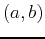has the equation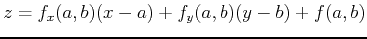. The following example will show you how this can easily be translated to Maple syntax.

> g := x-> sin(x)-x^3/7+x^2;
> tanline := D(g)(5)*(x-5)+g(5);
> plot({g(x),tanline},x=-2..8);


The next example shows how to find the tangent plane to the function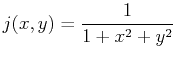at. You could write the partials with diff or D. This example uses D as it is easier to plug in the the point with this syntax; with diff the subs command would be used.

> j:=(x,y)->1/(1+x^2+y^2);
> tp:=D(j)(1/8,1/4)*(x-1/8)+D(j)(1/8,1/4)*(y-1/4)+j(1/8,1/4);
> plot3d({j(x,y),tp},x=-1..1,y=-1..1,style=patchnogrid);


To find a point where the tangent plane to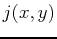is horizontal, you would need to solve where both first order partials are equal to zero simultaneously.

> solve({diff(j(x,y),x)=0,diff(j(x,y),y)=0},{x,y});


## Exercises

1. Compute the three distinct second order partial derivatives of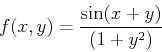at the pointusing the diff command and then again using the D command.

2. Given the single variable functiona)
Plotover the interval.
b)
Find the slope ofat.
c)
Find the two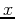values that have the same slope as in part b.
d)
For each of the points from part c, find the equation of the line that is tangent to the graph ofat these points. Plot the function and the tangent lines on the same graph over the interval given in part a to show the lines have the same slope.

3. Given: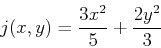a)
Find the tangent plane at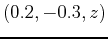.
b)
Plot the functionand the tangent plane on the same graph and rotate to see the point of tangency.
c)
Find the point on the graph ofwhere the tangent plane is horizontal. Plot the function and this tangent plane on the same graph and rotate to see the point of tangency.Next: About this document ... Up: lab_template Previous: lab_template
Dina J. Solitro-Rassias
2011-09-10